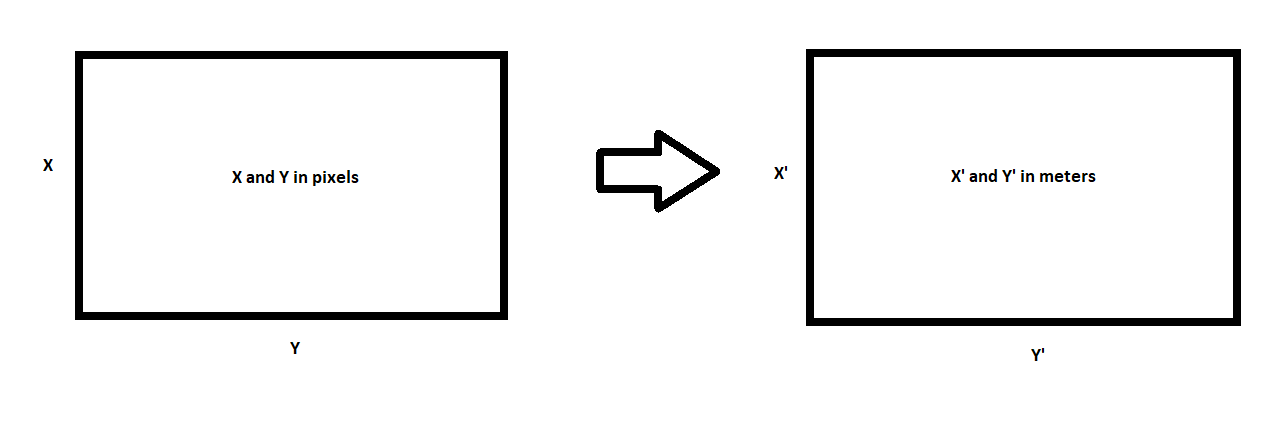# How to get the length and width of a frame of the video generated by the drone in meters?

#### jotarich

I have the flight log of the drone where I can get the height at the instant of time I want. Also, I have the length and width information in pixels of the frame. How can I calculate the length and width in meters?

•SkyFly

#### dirkclod

##### Moderator
Staff Member
No idea but someone can tell you I’m sure.
Welcome to the forum and is nice if you post in intros first
so we know a little about you before you jump in asking questions.•Harleydude and jotarich

#### Pygar70

Sorry, but your question doesn't make sense to me. Can you elaborate?

•Harleydude

#### jotarich

Sorry, but your question doesn't make sense to me. Can you elaborate?
Let's assume that an area covered by the drone was filmed at an A x B resolution (in pixels). From the flight log generated, I have the height at each instant of time of this recording. So I would like to know how to find the dimensions of a video frame in meters. I tried to make a drawing to try to help in understanding.•SkyFly

#### Meta4

Let's assume that an area covered by the drone was filmed at an A x B resolution (in pixels). From the flight log generated, I have the height at each instant of time of this recording. So I would like to know how to find the dimensions of a video frame in meters. I tried to make a drawing to try to help in understanding.
The answer to your question will only be dependent on the height of the camera above the ground and the aspect ratio of the image.
You will need to use a Field of View calculator like this one:

•DoomMeister and SkyFly

#### Andy9

Or simply calculate by the principles of geometry.

You have the width and length on the screen and you also have the distance from the ground, you calculate the size of the picture in the ground with the basic trigonometric function of the triangle.

•snowghost

#### MapMaker53

A bit easier (at least for people like me who are math challenged) would be to locate the 4 corners of your frame in Google Earth and add a location pin at each corner. Then simply use the measure tool to find the length and width of the captured ground in feet. This is assuming you shot your video straight down, An oblique view would produce different ground distances across the top and bottom of the frame.

•DonWiley and snowghost

#### Tiger64

Also need to factor in that drone is measuring height above takeoff point. If ground is sloping or up a hill or over a valley have more maths to do.

#### OzoneTech

There just do not exist sufficient data to calculate from pixel density and video dimension. One should use a 2D mapping software and use the phot geometry functions to calculate the dimensions.

#### Meta4

There just do not exist sufficient data to calculate from pixel density and video dimension. One should use a 2D mapping software and use the phot geometry functions to calculate the dimensions.
But it's a simple matter to find the field of view of the camera at any height.

#### OzoneTech

But it's a simple matter to find the field of view of the camera at any height.
Camera angle?

#### Meta4

Camera angle?
His post #4 suggests that the camera was shooting straight down.
Most people trying to work this out wouldn't be shooting at an oblique angle.

•Aairon

#### tribar

According to the P4P specs the horizontal Field Of View (FOV) is 84°.
Formula1: L = 2 x (h x tg(a/2))
Formula2: W = L / 16 x 9 (16:9 aspect ratio)
L=lenght, h=height, a=angle, W=width
tg(84/2)=0.9004
So at a height of 10 meters: L=18m & W=10.125m
CONDITIONS:
1. You're shooting (straight down) perpendicular to the ground.
2. The ground area is flat.
3. Height is from ground level, NOT take-off point.
Excel sheet:

•Pygar70, Aairon and JimD.

#### Meta4

According to the P4P specs the horizontal Field Of View (FOV) is 84°.
The angle of view for camera lenses is usually given as a diagonal, not horizontal angle.
The FoV for the P4 pro is 84° diagonally.

Another factor to be considered in answering the OP's question is we don't know what video resolution/aspect ratio he is using ... 4096×2160, 3840×2160 or 2720×1530.

•DoomMeister

#### Big_A

•DoomMeister